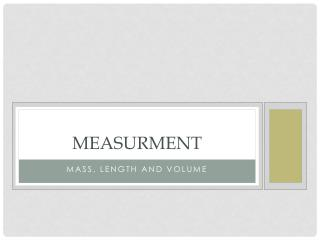# Measurment - PowerPoint PPT PresentationDownload PresentationMeasurment

MeasurmentDownload Presentation## Measurment

- - - - - - - - - - - - - - - - - - - - - - - - - - - E N D - - - - - - - - - - - - - - - - - - - - - - - - - - -
##### Presentation Transcript

1. Measurment Mass, Length and volume

2. Have you measured anything today? • Have you measured an object? • Have you measured an interval of time? • Have you measured the weight of an object? • How did you measure it?

3. Aims • Looking at Assumptions • Revisit units of length, volume and mass • Investigate how we measure these units

4. Basic Quantities • The unit of Length is the meter (m) • What are some multiples of this? • The unit of Area is meter squared (m2) • The unit of Volume is meters cubed (m3) • The unit of Mass is the kilogram (kg) • What are some multiples? • The unit of Time is seconds (s)

5. Measuring Objects • Using prior knowledge take the most accurate measurements of the following • How can we be more accurate?

6. Human Reaction Time • How do we measure time? • Reaction time concepts • Mental Processing time • Movement time • Device time • What are some instances where your reaction time can be very important? • How far does the ruler drop? • Did you improve with each try?